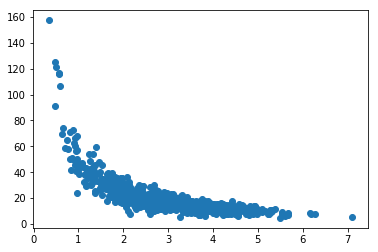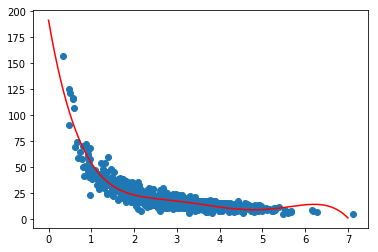# Polynomial Regression¶

Let's now take a look at some more real world data. For example page speed / purchase data:

In :
%matplotlib inline
from pylab import *
import numpy as np

np.random.seed(2)
pageSpeeds = np.random.normal(3.0, 1.0, 1000)
purchaseAmount = np.random.normal(50.0, 10.0, 1000) / pageSpeeds

scatter(pageSpeeds, purchaseAmount)

Out:
<matplotlib.collections.PathCollection at 0x7fd71cee26a0>Fortunately for us, python module numpy has a handy polyfit function that we can take advantage of. It allows us generate a nth-degree polynomial model of our data set that minimizes squared error. Let's try it with a 5th degree polynomial:

In :
x = np.array(pageSpeeds)
y = np.array(purchaseAmount)

p4 = np.poly1d(np.polyfit(x, y, 5))


We'll visualize our original scatter plot, together with a plot of our predicted values using the polynomial for page speed times ranging from 0-7 seconds:

In :
import matplotlib.pyplot as plt

xp = np.linspace(0, 7, 200)
plt.scatter(x, y)
plt.plot(xp, p4(xp), c='r')
plt.show()Looks pretty good! Let's measure the r-squared error:

In :
from sklearn.metrics import r2_score

r2 = r2_score(y, p4(x))

print(r2)

0.8553884386186101